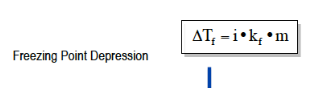# Problem: Assuming equal concentrations and complete dissociation, rank these aqueous solutions by their freezing points.Li2SO4NH4ICoCl3

###### FREE Expert Solution
94% (207 ratings)
###### FREE Expert Solution

Assuming that they have the same concentrations, the freezing point depression will depend on the van't Hoff factorWhere Tf is the freezing pt. depression; i as the van't hoff factor; Kf as the cryoscopic constant; and m as the molality

94% (207 ratings)###### Problem Details

Assuming equal concentrations and complete dissociation, rank these aqueous solutions by their freezing points.

Li2SO4

NH4I

CoCl3

What scientific concept do you need to know in order to solve this problem?

Our tutors have indicated that to solve this problem you will need to apply the The Colligative Properties concept. You can view video lessons to learn The Colligative Properties. Or if you need more The Colligative Properties practice, you can also practice The Colligative Properties practice problems.

What is the difficulty of this problem?

Our tutors rated the difficulty ofAssuming equal concentrations and complete dissociation, ran...as medium difficulty.

How long does this problem take to solve?

Our expert Chemistry tutor, Sabrina took 7 minutes and 2 seconds to solve this problem. You can follow their steps in the video explanation above.

What professor is this problem relevant for?

Based on our data, we think this problem is relevant for Professor Davis' class at UCF.